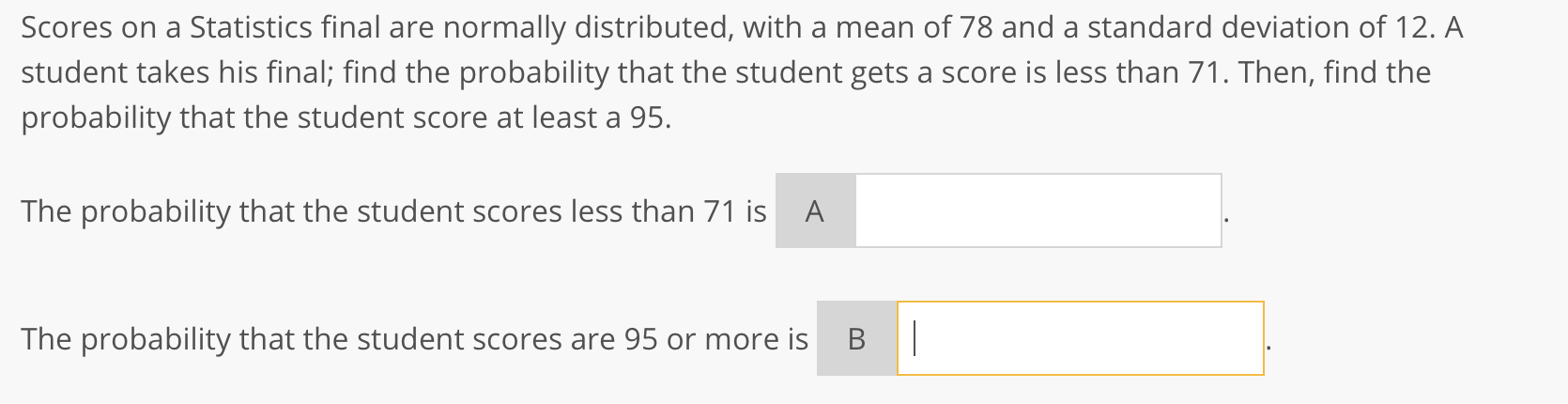# Question Solved1 AnswerScores on a Statistics final are normally distributed, with a mean of 78 and a standard deviation of 12. A student takes his final; find the probability that the student gets a score is less than 71. Then, find the probability that the student score at least a 95. The probability that the student scores less than 71 is A The probability that the student scores are 95 or more is BTG6QSDK The Asker · Probability and StatisticsTranscribed Image Text: Scores on a Statistics final are normally distributed, with a mean of 78 and a standard deviation of 12. A student takes his final; find the probability that the student gets a score is less than 71. Then, find the probability that the student score at least a 95. The probability that the student scores less than 71 is A The probability that the student scores are 95 or more is BT
More
Transcribed Image Text: Scores on a Statistics final are normally distributed, with a mean of 78 and a standard deviation of 12. A student takes his final; find the probability that the student gets a score is less than 71. Then, find the probability that the student score at least a 95. The probability that the student scores less than 71 is A The probability that the student scores are 95 or more is BT